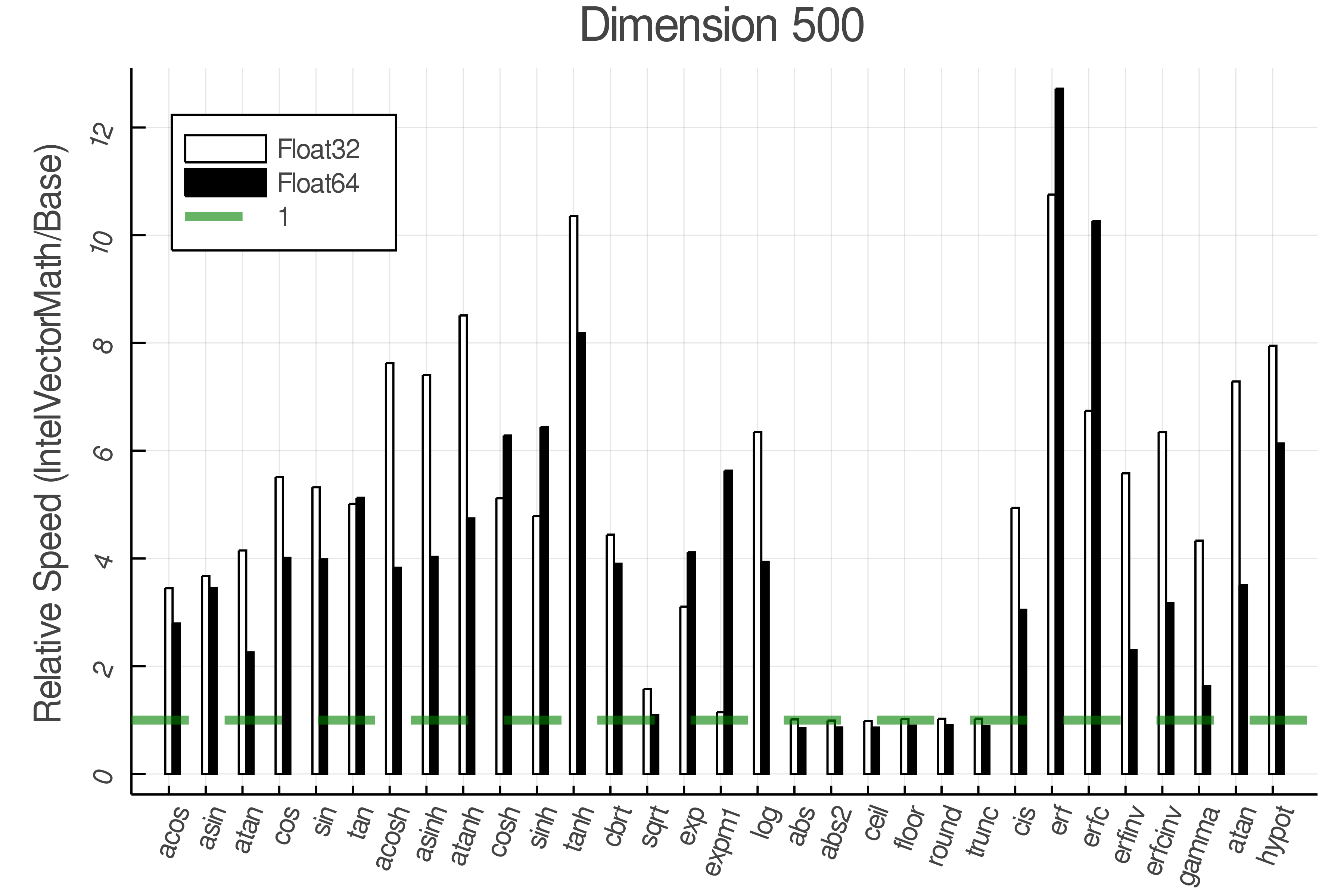## AcuteBenchmark.jl

Automatically benchmark functions!
Author aminya
Popularity
2 Stars
Updated Last
2 Years Ago
Started In
November 2019

# AcuteBenchmark

AcuteBenchmark allows you to benchmark functions that get Arrays as their input.

It is used inside IntelVectorMath for benchmarking its functions. A fully working example available here: https://github.com/JuliaMath/VML.jl/blob/AcuteBenchmark/benchmark/benchmark.jl

Creates random inputs for a function based on limits, types, and dims specified.

```config = Funb(
fun = sin,
limits = [(-1,1)],
types = [Float32, Float64],
dims = [10 100 200],
)```

or just in a compact form:

`config = Funb( sin, [(-1,1)], [Float32, Float64], [10 100 200])`
• fun: the function `:fun` or :(Module.fun)
• limits: min and max of possible values
• types : type of elements
• dims:
• each element gives the size of the input, and it is a:
• Number (for 1D)
• Tuple (for N-D)
• each row for each function argument
• each column for each dimension set.

use benchmark! to run the benchmark:

```using AcuteBenchmark

configs = FunbArray([
Funb( sin, [(-1,1)],[Float32, Float64],  );
Funb( atan, [(-1,1), (-1,1)],[Float32, Float64],[10; 10] );
Funb( *, [(-1, 1), (-1, 1), (-1, 1)], [Float32, Float64], [(10,10); (10,10)] );
])

benchmark!(configs)```

Plot the benchmark result using:

`bar(configs)`To have a same color for the same types use:

`bar(configs, uniqueType = true, dimAnnotation = true)`To plot the relative speed, pass a pair of configs:

`bar(configsRealBase => configsRealIVM, uniqueType = true, dimAnnotation = false, uniqueDim = true, "Base" => "IntelVectorMath")`To plot how the function acts over different dimension sets:

```configs2 = Funb( sin, [(-1,1)],[Float32, Float64], [10 30 50 100 200 500] );
benchmark!(configs2)
dimplot(configs2)```

The axes are logarithmic.To compare different sets pass an array of configs:

`dimplot([configsRealBase,configsRealIVM],["Base", "IntelVectorMath"])`

Use `AcuteBenchmark.save` and `AcuteBenchmark.load` to read and write the benchmark data.

```AcuteBenchmark.save("test.jld2", configs)